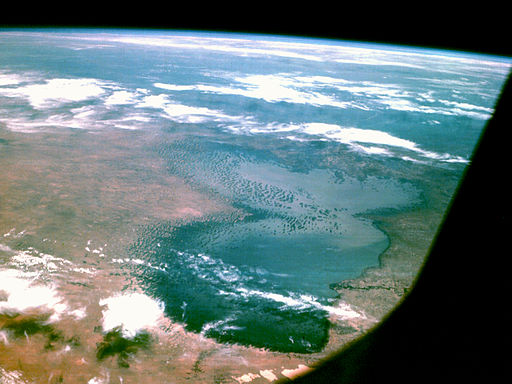# Using A Grid To Calculate The Area Of An Irregular Figure

Student View (Opens in new window)# Lesson Overview

Students estimate the area of Lake Chad by overlaying a grid on the map of the lake.

# Key Concept

The area of an irregular figure can be found by overlaying a grid on the figure. By estimating the number of grid squares the figure covers and multiplying by the area of each square, you can find the approximate area of the figure. The accuracy of the estimate depends on the size of the grid squares. Using a smaller grid leads to a more accurate estimate because more whole grid squares are completely filled. However, using a smaller grid also requires more counting and more combining of partially-filled squares and is, therefore, more time-consuming. Using a larger grid gives a quicker, but rougher, estimate of the area.

# Goals and Learning Objectives

• Use a grid to find the area of an irregular figure.

# Materials

• Map of Lake Chad handout (one for each pair of students)
• Rulers, optional (one for each pair of students)